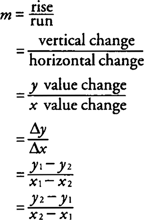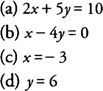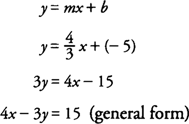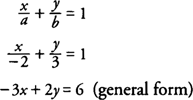## Linear Equations

A linear equation is any equation that can be expressed in the form ax + by = c, where a and b are not both zero. Although a linear equation may not be expressed in this form initially, it can be manipulated algebraically to this form. The slope of a line indicates whether the line slants up or down to the right or is horizontal or vertical. The slope is usually denoted by the letter m and is defined in a number of ways:Note that for a vertical line, the x value would remain constant, and the horizontal change would be zero; hence, a vertical line is said to have no slope or its slope is said to be nonexistent or undefined. All nonvertical lines have a numerical slope with a positive slope indicating a line slanting up to the right, a negative slope indicating a line slanting down to the right, and a slope of zero indicating a horizontal line.

Example 1: Find the slope of the line passing through (−5, 4) and (−1, −3).The line, then, has a slope of −7/4.

Some forms of expressing linear equations are given special names that identify how the equations are written. Note that even though these forms appear to be different from one another, they can be algebraically manipulated to show they are equivalent.

Any nonvertical lines are parallel if they have the same slopes, and conversely lines with equal slopes are parallel. If the slopes of two lines L 1 and L 2 are m 1 and m 2, respectively, then L 1 is parallel to L 2 if and only if m 1 = m 2.

Two nonvertical, nonhorizontal lines are perpendicular if the product of their slopes is −1, and conversely, if the product of their slopes is −1, the lines are perpendicular. If the slopes of two lines L 1 and L 2 are m 1 and m 2, respectively, then L 1 is perpendicular to L 2 if and only if m 1 · m 2 = −1.

You should note that any two vertical lines are parallel and a vertical line and a horizontal line are always perpendicular.

The general or standard form of a linear equation is ax + by = c, where a and b are not both zero. If b = 0, the equation takes the form x = constant and represents a vertical line. If a = 0, the equation takes the form y = constant and represents a horizontal line.

Example 2: The following are some examples of linear equations expressed in general form:The point‐slope form of a linear equation is yy 1 = m ( xx 1) when the line passes through the point ( x 1, y 1) and has a slope m.

Example 3: Find an equation of the line through the point (3,4) with slope −2/3.The slope‐intercept form of a linear equation is y = mx + b when the line has y‐intercept (0, b) and slope m.

Example 4: Find an equation of the line that has a slope 4/3 and crosses y‐axis at −5.The intercept form of a linear equation is x/a + y/b = 1 when the line has x‐intercept ( a,0) and y‐intercept (0, b).

Example 5: Find an equation of the line that crosses the x‐axis at −2 and the y‐axis at 3.